### Home > PC > Chapter 8 > Lesson 8.1.4 > Problem8-57

8-57.
1. Graph g(x) =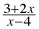. Homework Help ✎

1. Analyze the graph of g(x) and complete the following statements: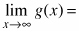____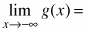____

2. Record both of these limit statements next to your graph.

3. Rewrite g(x) as a transformation of the parent graph y =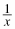.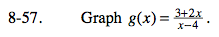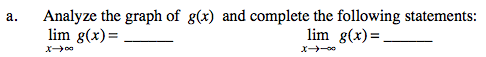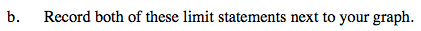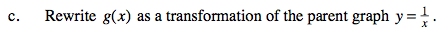$g(x)=\frac{3+2x}{x-4}=\frac{2(x-4)+11}{x-4}$